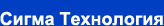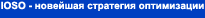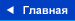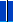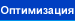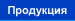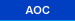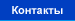### Методика сравнительного анализа методов оптимизации

 When evaluating the efficiency of optimization methods for different test problems it is necessary to find some “rule” how to take into account the “complexity” of the problem, or its “performances” – the number of design variables and constraints, their type (equality or inequality), the topological complexity of goal function and constraints. In our investigations we are used the following rule of this index evaluation.

##### Score = (10*Nx+5*Nineq+10*Neq)*SQRT[LOG(Error0/Error1)]/Ncalls, (***)
 where Error0 is starting Error, Error1 - final Error (zero values were replaced by 1e-16). Scores for each test problem were added for each algorithm, and then normalized by the highest total score. Next step is an evaluation of Comparative Score:

### CompScore=Score+K/R,

 K=1, if this task was solved succesfuly; K=0, if algorithm was failed (the algorithm did not improve Error value below 1e-2); R - rating according to score for this problem. The important place in optimization process is taken by the choice of search starting point. It can effect significantly upon the optimization process itself, and, consequently, upon the effectiveness indices when carrying out the comparative analysis. As known, most of the algorithms require starting point. However, there is a number of algorithms (IOSO algorithms are among them), which do not require this value. During the starting stage of extremum search these algorithms use the following hypothesis: ”I know that I know nothing about the researched object”. In practice usually it is known some basic solution, which can be assumed as the starting point. However, we can sight a number of real-life problems, for which the starting point and any information about the object is unavailable.

 (***) The main theses of such effectiveness evaluation approach were proposed by Oleg Golovinov.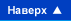© Сигма Технология 2001. E-mail: company@iosotech.com